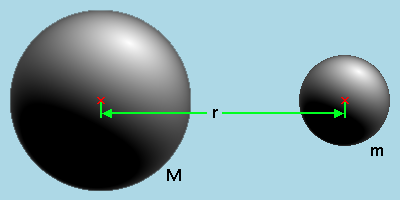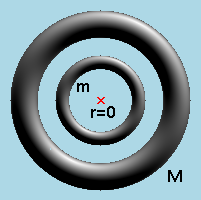# The new Newton-Confutation

And now follows a short confutation of the theory of gravitation by the greatest physicist of all time: Isaac Newton.In accordance with the theory of gravitation by Isaac Newton the mass of a body can be thought as united in the centre of gravity. The power of attraction on both balls in the picture above is then:

F = g*M*m/r²

Here M means the mass of the one body, m the mass of the other, r is the distance between the both centres of gravity and g is the "gravitational constant" with the strange unit: N*m²/kg². Therefore strange because nobody can imagine something about a mass to square. The "gravitational constant" shall be equal in the whole universe.

But the both bodies must not be balls, rings are allowed, too:Here everything stays the same.

F = g*M*m/r²

Of course you can vary the distance r.Ooops! What happened here? The distance of the both centres of gravity of the both rings is zero. And a division by zero is not allowed! (I know this very well, I am a programmer.) The power would be infinite. But in reality it is not.

Conclusion: Newton is confuted.

It is some kind of logic somehow. A force that is only attractive would agglutinate the whole universe. And thermic neutrons do not fall down either. And the planetary system would never be stable: a little change oft the speed of a planet would cause it to leave the sun-system or would make it bump into the sun immediately. Of the impossibility of the solution of the three bodies problem Henri Poincaré already knew to sing a song about.

And each atom of the universe had to know at each time, all the positions of all other atoms of the universe. The computer it would need would be bigger than the universe itself.

Saturn and Jupiter are spheric. After Newton their masses are lower than the mass of our air, therefore they are called gas-planets. But: if they exist out of gas they would be fogs like other fogs in the universe and not be that perfect spheric. And then they emanate more energy into the universe than they receive by the sun. But how should a cold gas gets this energy? And a hot gas would never be spheric. It seethes on the surface of the sun. And then Saturn and Jupiter have colours. But a gas should have been intermixed already. So where shall the colours come from?

Then Saturn, Jupiter, Uranus have rings exactly above the equator. But after Newton the equator is not specially distinguished.

To explain the agglutinating of the universe after Newton, monotheistic poled physicists need the big bang: the universe does agglutinate but not at once, first it has to get rid of the kinetic energy of the big bang. They find it conform with their book.

## How Newton developed his "Law of Gravitation"

Galileo found out, that a thrown stone in air follows the curve of a parabola. He could show, that this parabola is pieced by the superposition of two movements.

BombtrajectoryAlong the surface of the earth (in x-direction) the stone moves in air with a constant velocity and in the direction perpendicular to the surface of the earth the stone makes a consistent accelerated movement. The direction of the throw is a tangent on the bombtrajectory.

Now it was demanded from the mathematicians to find a theory that calculates to a curve the tangent at each point. This task was first solved by Newton and shortly later by Leibniz. Leibniz had no knowledge of the result of Newton, because as an alchemist, Newton kept his result secret for many years. But Newton became very fast as he realized that Leibniz has made the calculations public.

After Columbus substantiated after the Vikings once again, that the earth has the shape of a ball and Copernicus put the sun into the middle of the planetary system, the monotheists with their booklet had a problem. The then catholic Pontifex Maximus in Rome engaged through the emperor Rudolf II the protestant Kepler to confute the theory of Copernicus. Kepler first tinkered his own planetary system with the planets within the platonic bodies. After he realized that this was a nuisance, he quashed his own idea and found out, that if you take ellipses for the curves of the planets with the sun in one of the foci of the ellipses, then the Copernican world picture delivers better values than the Ptolemy one. With this the geocentric world picture was done.

Now the men of genius after Kepler had a tricky clout. It was asked to find a theory, that delivers in the result a Kepler ellipse. Newton succeed by the way, that he postulated a central force that goes with 1/r². For this he had to develop after the tangent mathematics the summation of infinite little parts because he needed for his central force a centre of gravity.

It is a circular reasoning if you substantiate out of the Kepler ellipses the gravitational force and then out of the gravitational force the elliptic shape of the curves of the planets.

Most students of physics do not realize this circular reasoning because they are employed enough by learning the mathematics (universalized 1/r**n potential with friction...).

Leibniz dissent Newton boldly. He accused Newton to open the door for occult speculation into science by postulating an only attractive force. What should that be a long-distant force that acts through a vacuum and this also without a loss of time?

Historic:
Neither Newton nor Leibniz can be seen as inventor of the infinitesimal calculus. Before them both Kepler showed 1615 in his Nova steriometria the way to calculate the volume of a ball. He imagined the volume of a ball reached by lots of cones with the height of the radius and with the spike in the middle of the ball and received so the volume of a ball as 1/3*surface*radius.

In the ancient Euclid and Archimedes had shown the calculation with infinite small parts with their methods of exhaustion. Euclid in his X. book and Archimedes found at the calculation of the area of a parabola the value reached by the progression 1/4 + (1/4)**2 + (1/4)**3 + ... + (1/4)**n = 1/3 arbitrary near.

By the way: the ancient Greeks like Platon wanted to ascribe the nature upon perfect objects. And perfect for them were balls and circles. So Ptolemaeus used circle (deferent and epicycle) to explain his world picture until Kepler said: the planet curves are ellipses. But what are ellipses? Well, a little circle that unrolls within a bigger circle: so circles, too. The deferent just has lost its middle. A theory must be easy and more easy than circles is not possible. The solution of the planetary curves might be found in the solution of the Hill's differential equation.